Question

# Write a balanced chemical equation for the dissociation of NH4Cl(s) into aqueous ions. Make sure to...

Write a balanced chemical equation for the dissociation of NH4Cl(s) into aqueous ions. Make sure to include heat in the equation as either a reactant or product.

NH4Cl (s) + Heat -----in water--------> NH4+(aq) + Cl-(aq)

NH4+(aq) + H2O(l) = NH3(aq) + H2O(l)

#### Earn Coins

Coins can be redeemed for fabulous gifts.

Similar Homework Help Questions
• ### Write a balanced chemical equation for the reaction of aqueous iron(III) ion with aqueous thiocyanate ion....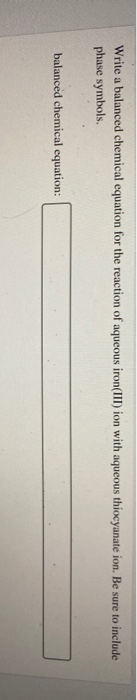Write a balanced chemical equation for the reaction of aqueous iron(III) ion with aqueous thiocyanate ion. Be sure to include phase symbols. balanced chemical equation:

• ### Write the balanced equation for the reaction of aqueous Pb(CIO), with aqueous Nal. Include phases. chemical...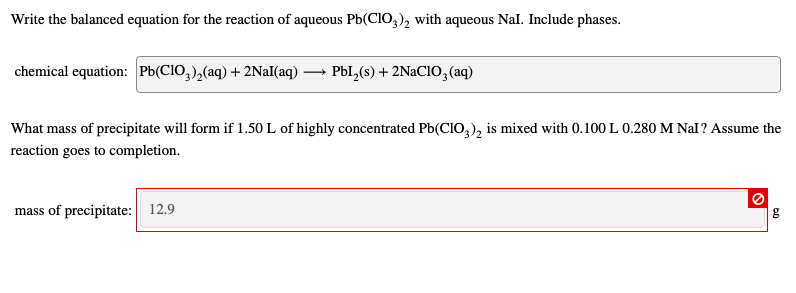Write the balanced equation for the reaction of aqueous Pb(CIO), with aqueous Nal. Include phases. chemical equation: Pb(ClO3)2(aq) + 2Nal(aq) — PbI(s) + 2NaCIO, (aq) What mass of precipitate will form if 1.50 L of highly concentrated Pb(CIO), is mixed with 0.100 L 0.280 M Nal? Assume the reaction goes to completion. mass of precipitate: 12.9

• ### Write the balanced equation for the reaction of aqueous Pb(CIO), with aqueous Nal. Include phases. chemical...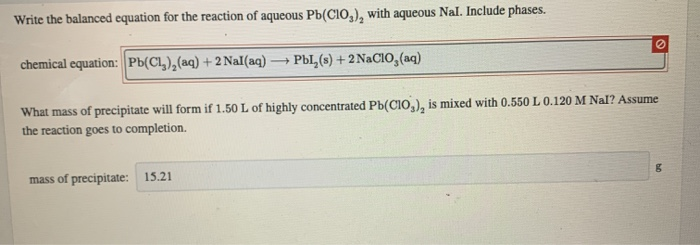Write the balanced equation for the reaction of aqueous Pb(CIO), with aqueous Nal. Include phases. chemical equation: Pb(Cl),(aq) + 2 Nal(aq) - PbI,(s) + 2 NaClO3(aq) What mass of precipitate will form if 1.50 L of highly concentrated Pb(CIO), is mixed with 0.550 L 0.120 M Nal? Assume the reaction goes to completion. mass of precipitate: 15.21

• ### ​Write a balanced chemical equation based on the following description: aqueous iron(III) chloride

Write a balanced chemical equation based on the following description: aqueous iron(III) chloride reacts with aqueous ammonium sulfide to make aqueous ammonium chloride and solid iron(III) sulfide

• ### Write a balanced equation for each of the processes in Part A. Remember to include heat...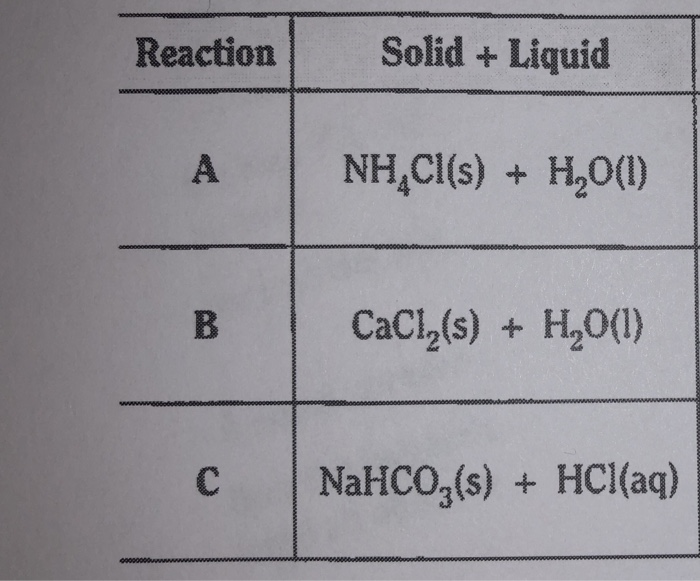Write a balanced equation for each of the processes in Part A. Remember to include heat on the reactant or product side, as appropiate. Reaction Solid + Liquid A NH,Cl(s) + H2O(1) CaCl (s) + H20(1) C NaHCO3(s) + HCl(aq) Reaction A B c Physical or Chemical Change? Chemical Chemical Chemical Exothermic or Endothermic? Endo Thermic Exothermic Endothermic

• ### what is the most common chemical method for removing H3O ions in aqueous solutions? write a...

what is the most common chemical method for removing H3O ions in aqueous solutions? write a balanced chemical equation to illustrate this procedure

• ### pitale contains lead, write the balanced chemical equation for this reaction. Be sure to include the...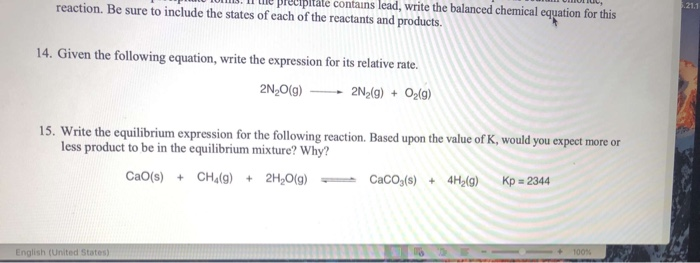pitale contains lead, write the balanced chemical equation for this reaction. Be sure to include the states of each of the reactants and products. 121.1 14. Given the following equation, write the expression for its relative rate. 2NO(9) 2N2(g) + O2(9) 15. Write the equilibrium expression for the following reaction. Based upon the value of K, would you expect more or less product to be in the equilibrium mixture? Why? CaO(s) + CH4(g) + 2H2O(g) Caco (s) + 4H2(g) Kp...

• ### Write the balanced equation for the reaction of aqueous Pb(ClO3)2 with aqueous Nal. Include phases. chemical equation:...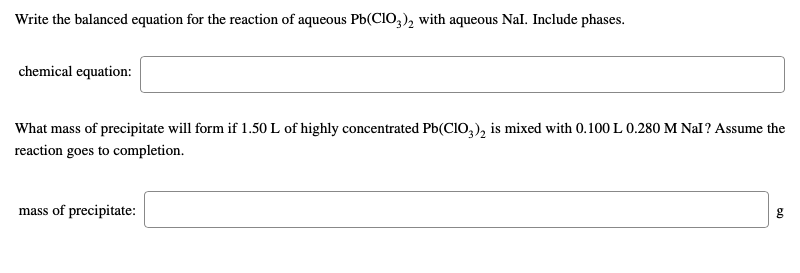Write the balanced equation for the reaction of aqueous Pb(ClO3)2 with aqueous Nal. Include phases. chemical equation: What mass of precipitate will form if 1.50 L of highly concentrated Pb(CIO), is mixed with 0.100 L 0.280 M Nal? Assume the reaction goes to completion. mass of precipitate:

• ### Write the balanced equation for the reaction of aqueous Pb(CIO), with aqueous Nal. Include phases. chemical...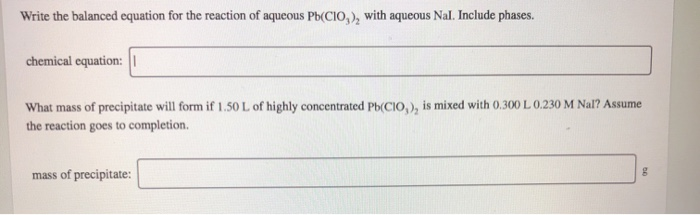Write the balanced equation for the reaction of aqueous Pb(CIO), with aqueous Nal. Include phases. chemical equation: What mass of precipitate will form if I 50 L of highly concentrated Pb(CIO), is mixed with 0.300 L 0.230 M Nal? Assume the reaction goes to completion. mass of precipitate:

• ### Write the balanced equation for the reaction of aqueous Pb(CIO3), with aqueous Nal. Include phases. chemical...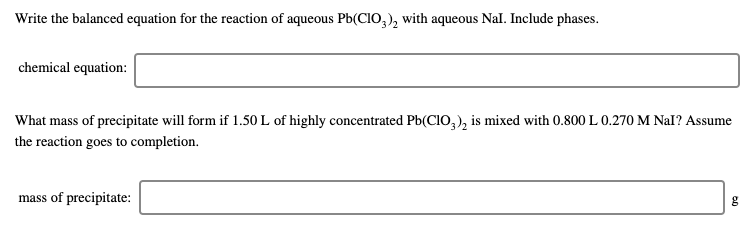Write the balanced equation for the reaction of aqueous Pb(CIO3), with aqueous Nal. Include phases. chemical equation: What mass of precipitate will form if 1.50 L of highly concentrated Pb(CIO,), is mixed with 0.800 L 0.270 M Nal? Assume the reaction goes to completion mass of precipitate: g